# Electrical engineering + fundamentals of Physics - math problems

#### Number of problems found: 30

• Cooker spiral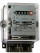A current of 4.4 A flow through the spiral of the electric cooker at a voltage of 220 V. What is the resistance of the spiral?
• Manganin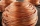How long must a manganin wire with a diameter of 0.8 mm have to have a value of 10Ω?
• Serial resistors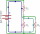In the circuit shown ( two resistiors R1 and R2 serial connected), the total resistance from A to B is 16 ohms. Find the resistance R2 and the current through the R1 = 12-Ohm resistor, if voltage between A and B is 10 V.
• Direction indicatorAs that which the electrical voltage is connected to the lamp direction indicator 7400 mOhm resistance when current of 1.6 A passes through it?
• The output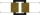The output voltage of the transformer is 880 V. The secondary coil has 1200 turns. Find the voltage to which the primary coil is connected and how many turns it has if a current of 1 A flows through it. Transformation ratio k = 4. What current flows throu
• The transformerThe primary coil of the transformer has 1100 turns and is connected to a voltage of 220 V. How many turns does the secondary coil have when it has a voltage of 55 V? Determine the transformation ratio and decide what the transformation is.
• Circuit voltageCalculate the closed-circuit voltage. Calculate the power on the resistors. R1 = 30 ohms, R2 = 10 ohms. I = 0.1A. U =?. The resistors are connected in series, one after the other.
• The resistanceWhat is the resistance of an aluminum wire 0.2 km long and 10 mm in diameter?
• Copper windingCalculate the current flowing through the copper winding at an operating temperature of 70°C. Celsius, if the winding diameter is 1.128 mm and the winding length is 40 m. The winding is connected to 8V.
• Current in the conductorCalculate the current in the conductor (in mA) if it is connected to a 4.5 V voltage source and its resistance is 20 (ohm).
• The bulbThe bulb has a reading of 6V-0.05 A, what current flows through the bulb if we connect it to a cell with a voltage of 12V?
• Electric workCalculate the work done by the electric forces passing the current of 0.2 A through the bulb in 10 minutes if the bulb is connected to a 230 V power supply.
• Two resistorsTwo resistors 20 Ω and 60 Ω are connected in series and an external voltage of 400 V is connected to them. What are the electrical voltages on the respective resistors? Please comment!
• Lowest voltage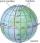Three resistors with resistors R1 = 10 kΩ, R2 = 20 kΩ, R3 = 30 kΩ are connected in series and an external voltage U = 30 V is connected to them. On which resistor is the lowest voltage?
• Copper Cu wireCopper wire with a diameter of 1 mm and a weight of 350 g is wound on a spool. Calculate its length if the copper density is p = 8.9 g/cm cubic.
• Cooker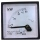A current of 2A passes through the immersion cooker at a voltage of 230V. What work do the electric field forces in 2 minutes?
• The copper wireThe copper wire bundle with a diameter of 2.8mm has a weight of 5kg. How many meters of wire is bundled if 1m3 of copper weighs 8930kg?
• Voltmeter range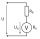We have a voltmeter which in the original set measures voltage to 10V. Calculate the size of the ballast resistor for this voltmeter, if we want to measure the voltage up to 50V. Voltmeter's internal resistance is 2 kiloohm/Volt.
• Orlík hydroelectric plantThe Orlík hydroelectric power plant, built in 1954-1961, consists of four Kaplan turbines. For each of them, the water with a flow rate of Q = 150 m3/s is supplied with a flow rate of h = 70.5 m at full power. a) What is the total installed power of the p
• TransformerSolve the textbook problems - transformer: a) N1 = 40, N2 = 80, U2 = 80 V, U1 =? b) N1 = 400, U1 = 200 V, U2 = 50 V, N2 =?

Do you have an exciting math question or word problem that you can't solve? Ask a question or post a math problem, and we can try to solve it.

We will send a solution to your e-mail address. Solved examples are also published here. Please enter the e-mail correctly and check whether you don't have a full mailbox.

Electrical engineering - math problems. Fundamentals of Physics - math problems.